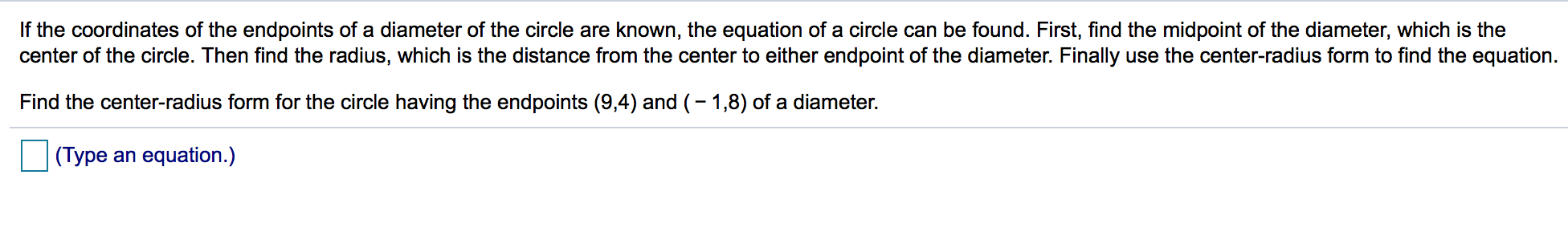# If the coordinates of the endpoints of a diameter of the circle are known, the equation of a circle can be found. First, find the midpoint of the diameter, which is the center of the circle. Then find the radius, which is the distance from the center to either endpoint of the diameter. Finally use the center-radius form to find the equation. Find the center-radius form for the circle having the endpoints (9,4) and (-1,8) of a diameter. (Type an equation.)

Questionhelp_outlineImage TranscriptioncloseIf the coordinates of the endpoints of a diameter of the circle are known, the equation of a circle can be found. First, find the midpoint of the diameter, which is the center of the circle. Then find the radius, which is the distance from the center to either endpoint of the diameter. Finally use the center-radius form to find the equation. Find the center-radius form for the circle having the endpoints (9,4) and (-1,8) of a diameter. (Type an equation.) fullscreen